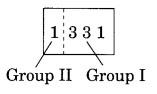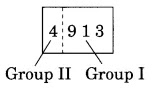NCERT Solutions for Class 8 Maths Chapter 7 Cubes and Cube Roots Ex 7.2

# NCERT Solutions for Class 8 Maths Chapter 7 Cubes and Cube Roots Ex 7.2

## NCERT Solutions for Class 8 Maths Chapter 7 Cubes and Cube Roots Ex 7.2

NCERT Solutions for Class 8 Maths Chapter 7 Cubes and Cube Roots Ex 7.2 are the part of NCERT Solutions for Class 8 Maths. Here you can find the NCERT Solutions for Class 8 Maths Chapter 7 Cubes and Cube Roots Ex 7.2.

### Ex 7.2 Class 8 Maths Question 1.

Find the cube root of each of the following numbers by prime factorisation method.
(i) 64
(ii) 512
(iii) 10648
(iv) 27000
(v) 15625
(vi) 13824
(vii) 110592
(viii) 46656
(ix) 175616
(x) 91125

Solution:

### Ex 7.2 Class 8 Maths Question 2.

State true or false.
(i) Cube of any odd number is even.
(ii) A perfect cube does not end with two zeros.
(iii) If the square of a number ends with 5, then its cube ends with 25.
(iv) There is no perfect cube which ends with 8.
(v) The cube of a two digit number may be a three digit number.
(vi) The cube of a two digit number may have seven or more digits.

(vii) The cube of a single digit number may be a single digit number.

Solution:
(i) False – Because the cube of any odd number is always odd, e.g., (5)3 = 125
(ii) True – Because a perfect cube does not end with two zeros.
(iii) True – Because if the square of a number ends with 5, then its cube ends with 25, e.g., (5)2 = 25 and (5)3 = 625
(iv) False – Because (12)3 = 1728 (ends with 8)
(v) False – Because (10)3 = 1000 (4-digit number)
(vi) False – Because (99)3 = 970299 (6-digit number)
(vii) True – Because (2)3 = 8 (1-digit number)

### Ex 7.2 Class 8 Maths Question 3.

You are told that 1,331 is a perfect cube. Can you guess without factorisation what is its cube root? Similarly, guess the cube roots of 4913, 12167, 32768.

Solution:
The given perfect cube = 1331
Forming groups of three from the rightmost digits, we get2nd group = 1
1st group = 331
Ones digit in first group = 1
So, ones digit in the required cube root may be 1.
The second group has only 1.
The estimated cube root of 1331 = 11
Thus, 31331 = 11
(i) The given perfect cube = 4913
Forming groups of three from the rightmost digit, we get2nd group = 4
1st group = 913
Ones digit in 913 is 3.
So, ones digit in the cube root of the given number may be 7.
Now, in 2nd group, the digit is 4.
13 < 4 < 23
Therefore, the tens must be the smallest number 1.
Thus, the estimated cube root of 4913 = 17.
(ii) The given perfect cube = 12167
Forming groups of three from the rightmost digit, we get2nd group = 12
1st group = 167
The ones digit in 167 is 7.
So, ones digit in the cube root of the given number may be 3.
Now, in 2nd group, we have 12
23 < 12 < 33
Therefore, the tens digit of the required cube root of the given number is 2.
Thus, the estimated cube root of 12167 is 23.
(iii) The given perfect cube = 32768
Forming groups of three from the rightmost digit, we get2nd group = 32
1st group = 768
Ones digit in 768 is 8.
So, ones digit in the cube root of the given number may be 2.
Now, in 2nd group, we have 32.
33 < 32 < 43
Therefore, the tens digit of the cube root of the given number is 3.
Thus, the estimated cube root of 32768 is 32.

You can also like these:

NCERT Solutions for Maths Class 9

NCERT Solutions for Maths Class 10

NCERT Solutions for Maths Class 11

NCERT Solutions for Maths Class 12